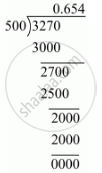# Express the Following Rational Number as Decimal: 327/500 - Mathematics

Express the following rational number as decimal:

327/500

#### Solution

Given rational number is 327/500

Now we have to express this rational number into decimal form. So we will use long division method as below.Hence, 327/500=0.654

Concept: Introduction of Real Number
Is there an error in this question or solution?
Chapter 1: Number Systems - Exercise 1.2 [Page 13]

#### APPEARS IN

RD Sharma Mathematics for Class 9
Chapter 1 Number Systems
Exercise 1.2 | Q 1.2 | Page 13
Share